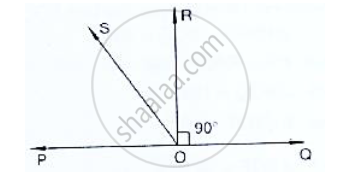# 19. In the Below Fig, Poq is a Line. Ray Or is Perpendicular to Line Pq. Os is Another Ray Lying Between Rays Op and Or. Prove that ∠Ros = 1 (∠Qos − ∠Pos). - Mathematics

In the below fig, POQ is a line. Ray OR is perpendicular to line OS is another ray lying

between rays OP and OR. Prove that ∠ROS = 1 (∠QOS − ∠POS).#### Solution

Given that, OR perpendicular

∴∠POR = 90°

∠POS + ∠SOR = 90°                 [∴ ∠POR = ∠POS + ∠SOR]

∠ROS = 90° - ∠POS         ..........(1)

∠QOR = 90°           (∴ OR  ⊥ PQ)

∠QOS - ∠ROS = 90°

∠ROS = ∠QOS - 90°             .........(2)

By adding (1) and (2) equations, we get

2∠ROS = ∠QOS - ∠POS

∠ROS = 1/2 (∠QOS - ∠POS )

Concept: Introduction to Lines and Angles
Is there an error in this question or solution?

#### APPEARS IN

RD Sharma Mathematics for Class 9
Chapter 10 Lines and Angles
Exercise 10.2 | Q 19 | Page 17